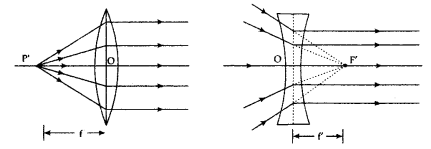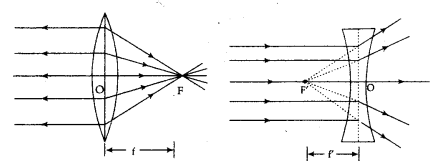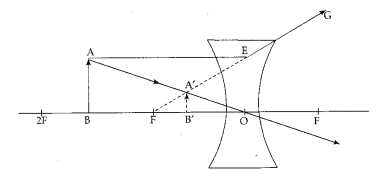# (a) Define focal length of a spherical lens

(a) Define focal length of a spherical lens.
(b) A divergent lens has a focal length of 30 cm. At what distance should an object of height 5 cm from the optical centre of the lens be placed so that its image is formed 15 cm away from the lens? Find the size of the image also.
Draw a ray diagram to show the formation of image in the above situation.

1. Principal focal length:
First principal focus and first focal length: It is a fixed point on the principal axis such that rays starting from this point (in convex lens) or appearing to go towards this point (concave lens) after refraction through the lens, become parallel to the principal axis. It is represented but F1 or f. The plane passing through this point and perpendicular to the principal axis is called the first focal plane. The distance between first principal focus and the optical centre is called the first focal length. It is denoted by f1 or f’.Second principal focus and second focal length: It is a fixed point on the principal axis such that the light rays incident parallel to the principal axis after refraction through the lens, either converge to this point (in convex lens) or appear to diverge from this point (in concave lens). The plane passing through this point and perpendicular to principal axis is called the second focal plane. The distance between the second principal focus and the optical centre is called the second focal length. It is denoted by f2 and f1. Given: divergent lens (concave lens)1.